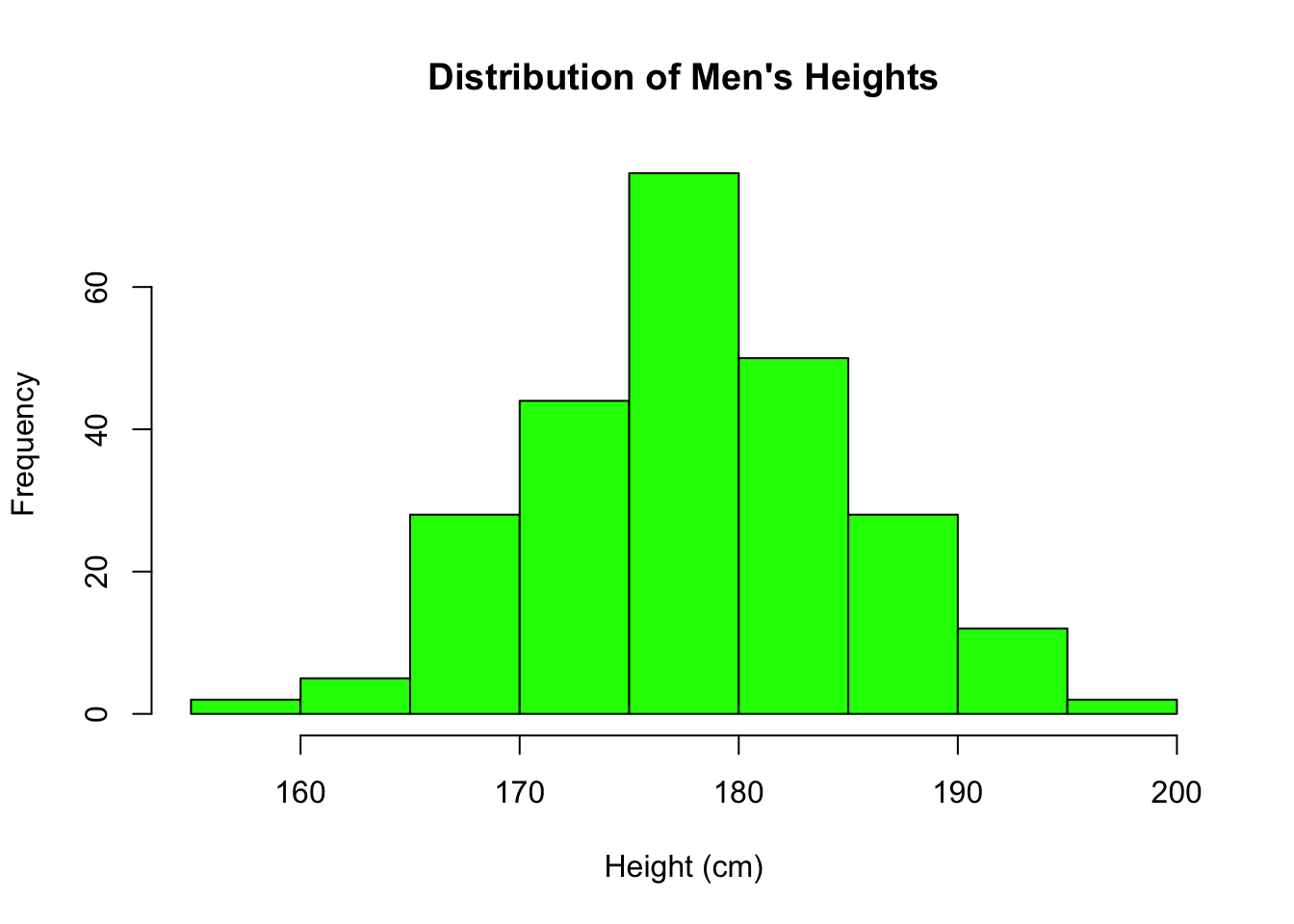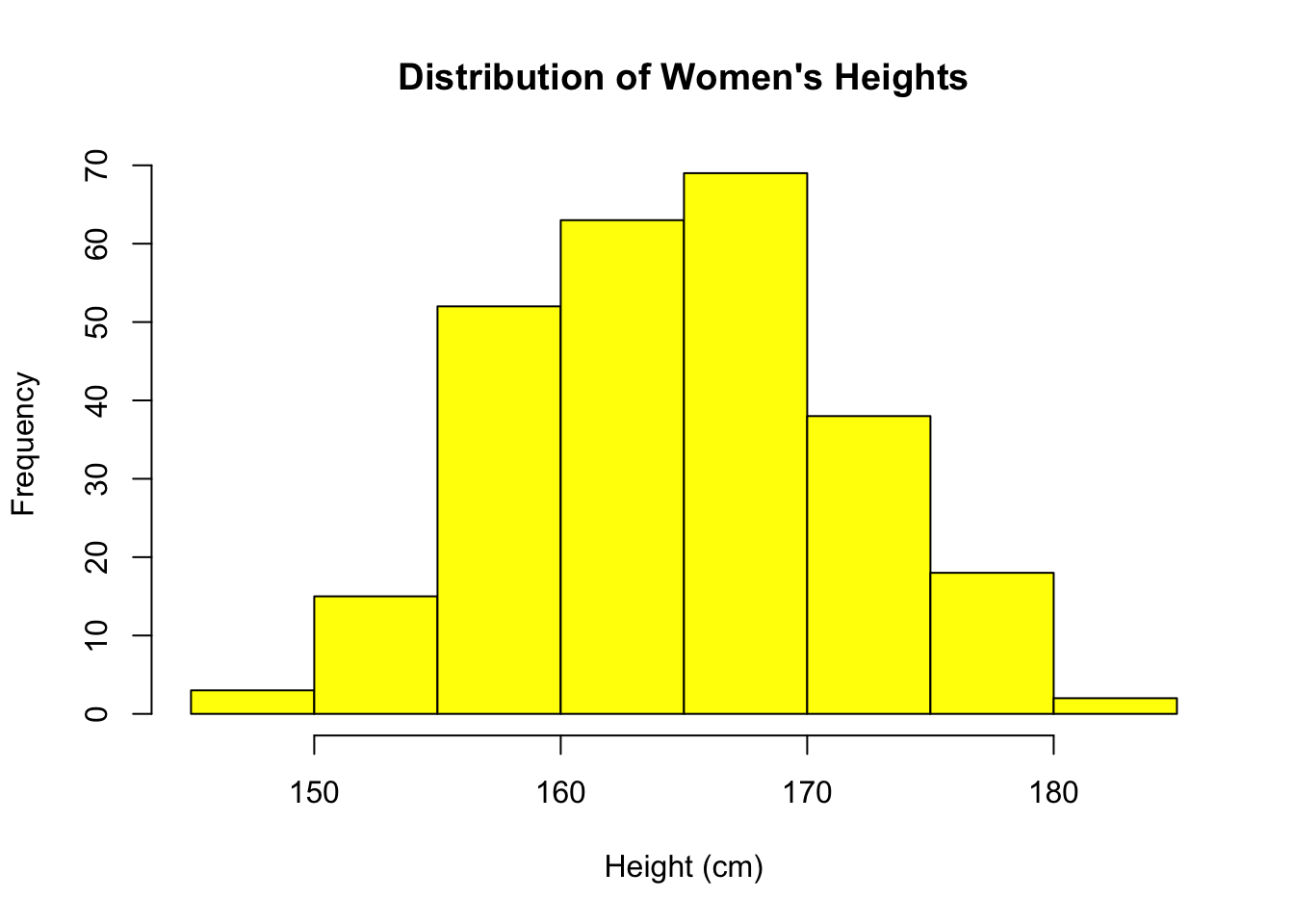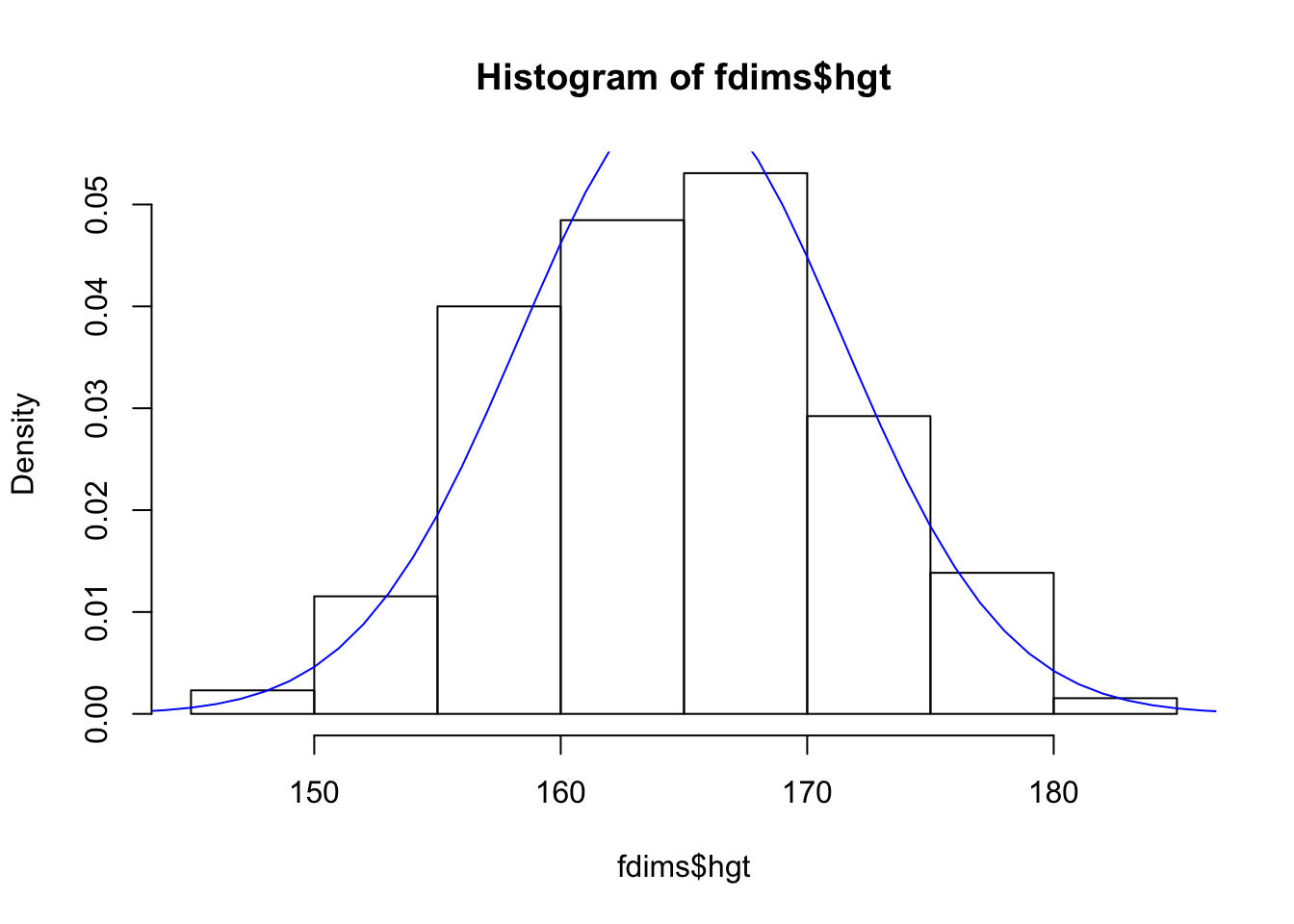In this lab we’ll investigate the probability distribution that is most central to statistics: the normal distribution. If we are confident that our data are nearly normal, that opens the door to many powerful statistical methods. Here we’ll use the graphical tools of R to assess the normality of our data and also learn how to generate random numbers from a normal distribution.

## The Data

This week we’ll be working with measurements of body dimensions. This data set contains measurements from 247 men and 260 women, most of whom were considered healthy young adults.

``load("more/bdims.RData")``

Let’s take a quick peek at the first few rows of the data.

``head(bdims)``
``````##   bia.di bii.di bit.di che.de che.di elb.di wri.di kne.di ank.di sho.gi
## 1   42.9   26.0   31.5   17.7   28.0   13.1   10.4   18.8   14.1  106.2
## 2   43.7   28.5   33.5   16.9   30.8   14.0   11.8   20.6   15.1  110.5
## 3   40.1   28.2   33.3   20.9   31.7   13.9   10.9   19.7   14.1  115.1
## 4   44.3   29.9   34.0   18.4   28.2   13.9   11.2   20.9   15.0  104.5
## 5   42.5   29.9   34.0   21.5   29.4   15.2   11.6   20.7   14.9  107.5
## 6   43.3   27.0   31.5   19.6   31.3   14.0   11.5   18.8   13.9  119.8
##   che.gi wai.gi nav.gi hip.gi thi.gi bic.gi for.gi kne.gi cal.gi ank.gi
## 1   89.5   71.5   74.5   93.5   51.5   32.5   26.0   34.5   36.5   23.5
## 2   97.0   79.0   86.5   94.8   51.5   34.4   28.0   36.5   37.5   24.5
## 3   97.5   83.2   82.9   95.0   57.3   33.4   28.8   37.0   37.3   21.9
## 4   97.0   77.8   78.8   94.0   53.0   31.0   26.2   37.0   34.8   23.0
## 5   97.5   80.0   82.5   98.5   55.4   32.0   28.4   37.7   38.6   24.4
## 6   99.9   82.5   80.1   95.3   57.5   33.0   28.0   36.6   36.1   23.5
##   wri.gi age  wgt   hgt sex
## 1   16.5  21 65.6 174.0   1
## 2   17.0  23 71.8 175.3   1
## 3   16.9  28 80.7 193.5   1
## 4   16.6  23 72.6 186.5   1
## 5   18.0  22 78.8 187.2   1
## 6   16.9  21 74.8 181.5   1``````

You’ll see that for every observation we have 25 measurements, many of which are either diameters or girths. A key to the variable names can be found at http://www.openintro.org/stat/data/bdims.php, but we’ll be focusing on just three columns to get started: weight in kg (`wgt`), height in cm (`hgt`), and `sex` (`1` indicates male, `0` indicates female).

Since males and females tend to have different body dimensions, it will be useful to create two additional data sets: one with only men and another with only women.

``````mdims <- subset(bdims, sex == 1)
fdims <- subset(bdims, sex == 0)``````
1. Make a histogram of men’s heights and a histogram of women’s heights. How would you compare the various aspects of the two distributions?

The histogram of men’s heights is a normal unimodal distribution centered at 175-180cm.

``````hist(mdims\$hgt,
main="Distribution of Men's Heights",
xlab="Height (cm)",
col="green")``````The histogram of women’s heights is a left-skewed unimodal distribution centered at 165cm

``````hist(fdims\$hgt,
main="Distribution of Women's Heights",
xlab="Height (cm)",
col="yellow")``````## The normal distribution

In your description of the distributions, did you use words like bell-shaped or normal? It’s tempting to say so when faced with a unimodal symmetric distribution.

To see how accurate that description is, we can plot a normal distribution curve on top of a histogram to see how closely the data follow a normal distribution. This normal curve should have the same mean and standard deviation as the data. We’ll be working with women’s heights, so let’s store them as a separate object and then calculate some statistics that will be referenced later.

``````fhgtmean <- mean(fdims\$hgt)
fhgtsd   <- sd(fdims\$hgt)``````

Next we make a density histogram to use as the backdrop and use the `lines` function to overlay a normal probability curve. The difference between a frequency histogram and a density histogram is that while in a frequency histogram the heights of the bars add up to the total number of observations, in a density histogram the areas of the bars add up to 1. The area of each bar can be calculated as simply the height times the width of the bar. Using a density histogram allows us to properly overlay a normal distribution curve over the histogram since the curve is a normal probability density function. Frequency and density histograms both display the same exact shape; they only differ in their y-axis. You can verify this by comparing the frequency histogram you constructed earlier and the density histogram created by the commands below.

``````hist(fdims\$hgt, probability = TRUE)
x <- 140:190
y <- dnorm(x = x, mean = fhgtmean, sd = fhgtsd)
lines(x = x, y = y, col = "blue")``````After plotting the density histogram with the first command, we create the x- and y-coordinates for the normal curve. We chose the `x` range as 140 to 190 in order to span the entire range of `fheight`. To create `y`, we use `dnorm` to calculate the density of each of those x-values in a distribution that is normal with mean `fhgtmean` and standard deviation `fhgtsd`. The final command draws a curve on the existing plot (the density histogram) by connecting each of the points specified by `x` and `y`. The argument `col` simply sets the color for the line to be drawn. If we left it out, the line would be drawn in black.

The top of the curve is cut off because the limits of the x- and y-axes are set to best fit the histogram. To adjust the y-axis you can add a third argument to the histogram function: `ylim = c(0, 0.06)`.

1. Based on the this plot, does it appear that the data follow a nearly normal distribution?

Yes, it appears like the data are somewhat close to a normal distribution.

## Evaluating the normal distribution

Eyeballing the shape of the histogram is one way to determine if the data appear to be nearly normally distributed, but it can be frustrating to decide just how close the histogram is to the curve. An alternative approach involves constructing a normal probability plot, also called a normal Q-Q plot for “quantile-quantile”.

``````qqnorm(fdims\$hgt)
qqline(fdims\$hgt)``````